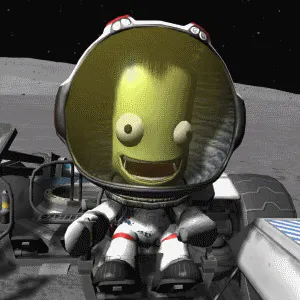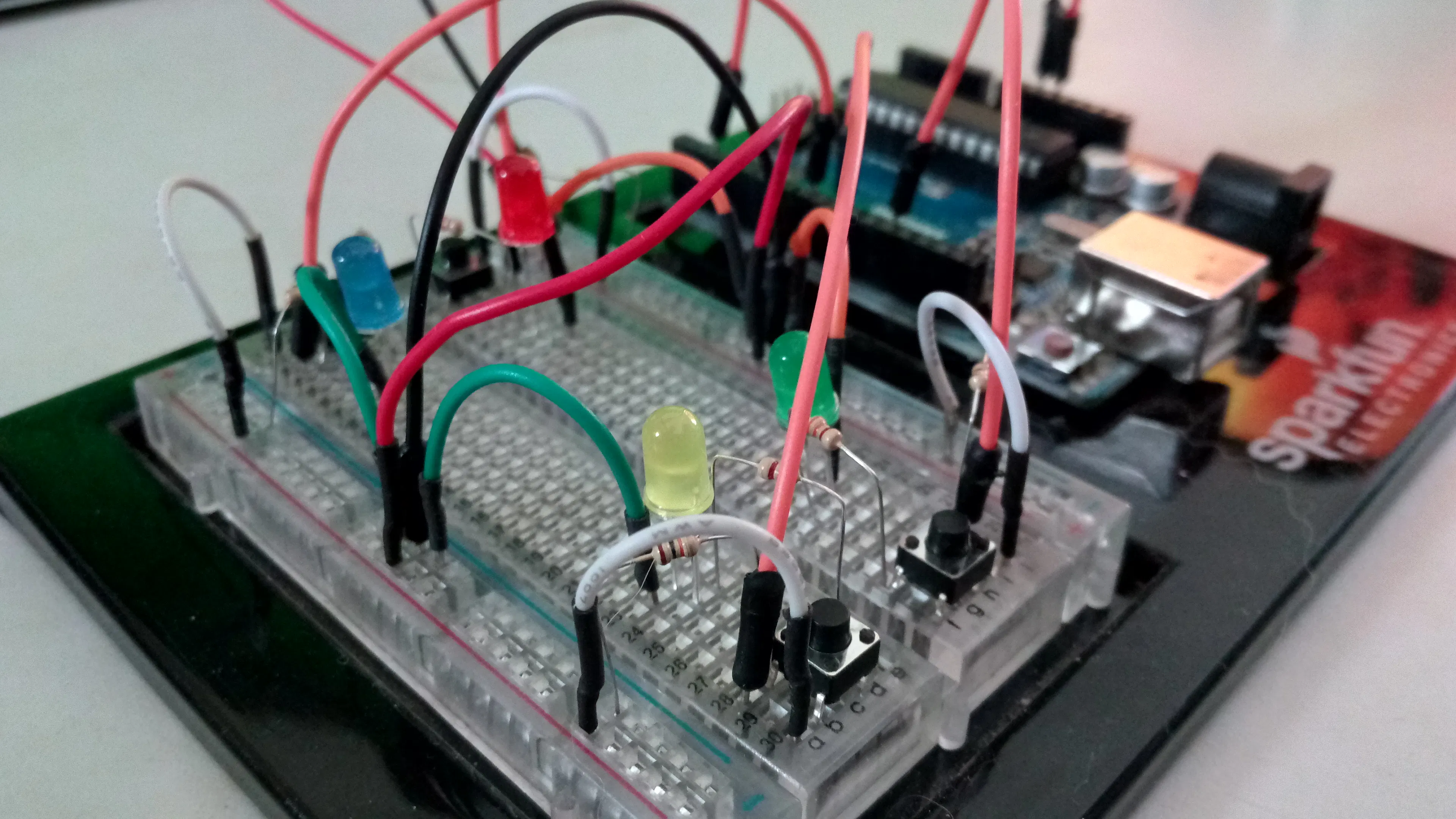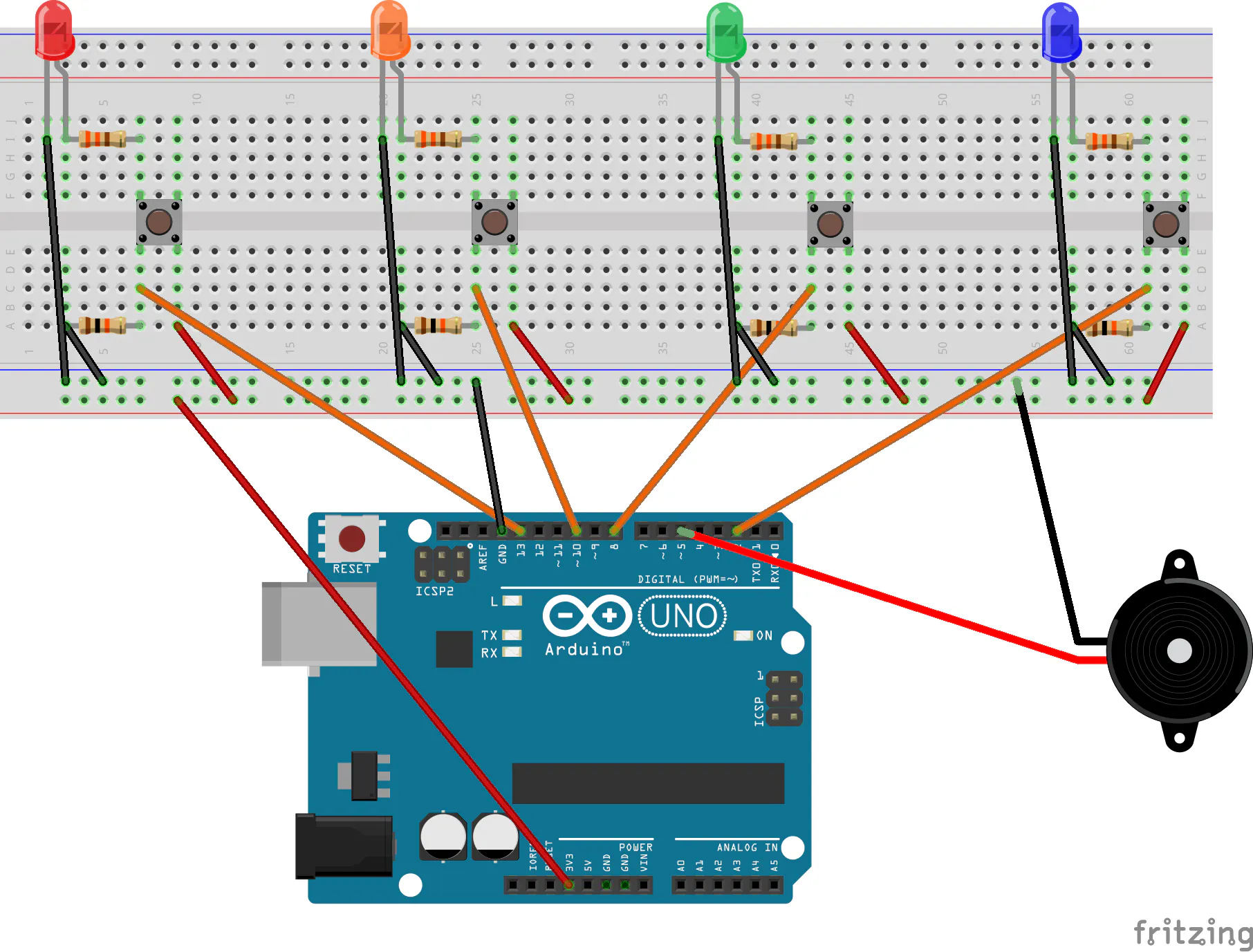# Make an Arduino Memory Game

A tutorial for beginners for making a memory game with an arduino and leds

BeginnerFull instructions provided24,652## Things used in this project

### Hardware componentsArduino UNO & Genuino UNO
×1
 SparkFun 330 ohm resistors we need 4 of them only
×1
 SparkFun 10k ohm resistors we need 4 of them only
×1
 SparkFun Assorted LEDs we need 4 LEDs of different colors
×1
 SparkFun Mini speaker You can do without this if you don't care about the sound
×1
×1Jumper wires (generic)
×1

### Software apps and online servicesArduino IDE

## Schematics

### Diagram## Code

### Memory Game Code

Arduino
This is the entire code for the game. Simply copy and paste it in your favorite IDE and upload it to the Arduino.
```#define PLAYER_WAIT_TIME 2000 // The time allowed between button presses - 2s

byte sequence;           // Storage for the light sequence
byte curLen = 0;              // Current length of the sequence
byte inputCount = 0;          // The number of times that the player has pressed a (correct) button in a given turn
byte lastInput = 0;           // Last input from the player
byte expRd = 0;               // The LED that's suppose to be lit by the player
bool btnDwn = false;          // Used to check if a button is pressed
bool wait = false;            // Is the program waiting for the user to press a button
bool resetFlag = false;       // Used to indicate to the program that once the player lost

byte soundPin = 5;            // Speaker output

byte noPins = 4;              // Number of buttons/LEDs (While working on this, I was using only 2 LEDs)
// You could make the game harder by adding an additional LED/button/resistors combination.
byte pins[] = {2, 13, 10, 8}; // Button input pins and LED ouput pins - change these vaules if you wwant to connect yourbuttons to other pins
// The number of elements must match noPins below

long inputTime = 0;           // Timer variable for the delay between user inputs

void setup() {
delay(3000);                // This is to give me time to breathe after connection the arduino - can be removed if you want
Serial.begin(9600);         // Start Serial monitor. This can be removed too as long as you remove all references to Serial below
Reset();
}

///
/// Sets all the pins as either INPUT or OUTPUT based on the value of 'dir'
///
void setPinDirection(byte dir){
for(byte i = 0; i < noPins; i++){
pinMode(pins[i], dir);
}
}

//send the same value to all the LED pins
void writeAllPins(byte val){
for(byte i = 0; i < noPins; i++){
digitalWrite(pins[i], val);
}
}

//Makes a (very annoying :) beep sound
void beep(byte freq){
analogWrite(soundPin, 2);
delay(freq);
analogWrite(soundPin, 0);
delay(freq);
}

///
/// Flashes all the LEDs together
/// freq is the blink speed - small number -> fast | big number -> slow
///
void flash(short freq){
setPinDirection(OUTPUT); /// We're activating the LEDS now
for(int i = 0; i < 5; i++){
writeAllPins(HIGH);
beep(50);
delay(freq);
writeAllPins(LOW);
delay(freq);
}
}

///
///This function resets all the game variables to their default values
///
void Reset(){
flash(500);
curLen = 0;
inputCount = 0;
lastInput = 0;
expRd = 0;
btnDwn = false;
wait = false;
resetFlag = false;
}

///
/// User lost
///
void Lose(){
flash(50);
}

///
/// The arduino shows the user what must be memorized
/// Also called after losing to show you what you last sequence was
///
void playSequence(){
//Loop through the stored sequence and light the appropriate LEDs in turn
for(int i = 0; i < curLen; i++){
Serial.print("Seq: ");
Serial.print(i);
Serial.print("Pin: ");
Serial.println(sequence[i]);
digitalWrite(sequence[i], HIGH);
delay(500);
digitalWrite(sequence[i], LOW);
delay(250);
}
}

///
/// The events that occur upon a loss
///
void DoLoseProcess(){
Lose();             // Flash all the LEDS quickly (see Lose function)
delay(1000);
playSequence();     // Shows the user the last sequence - So you can count remember your best score - Mine's 22 by the way :)
delay(1000);
Reset();            // Reset everything for a new game
}

///
/// Where the magic happens
///
void loop() {
if(!wait){
//****************//
// Arduino's turn //
//****************//
setPinDirection(OUTPUT);                      // We're using the LEDs

sequence[curLen] = pins[random(0,noPins)];    // Put a new random value in the next position in the sequence -  https://www.arduino.cc/en/Reference/random
curLen++;                                     // Set the new Current length of the sequence

playSequence();                               // Show the sequence to the player
beep(50);                                     // Make a beep for the player to be aware

wait = true;                                  // Set Wait to true as it's now going to be the turn of the player
inputTime = millis();                         // Store the time to measure the player's response time
}else{
//***************//
// Player's turn //
//***************//
setPinDirection(INPUT);                       // We're using the buttons

if(millis() - inputTime > PLAYER_WAIT_TIME){  // If the player takes more than the allowed time,
DoLoseProcess();                            // All is lost :(
return;
}

if(!btnDwn){                                  //
expRd = sequence[inputCount];               // Find the value we expect from the player
Serial.print("Expected: ");                 // Serial Monitor Output - Should be removed if you removed the Serial.begin above
Serial.println(expRd);                      // Serial Monitor Output - Should be removed if you removed the Serial.begin above

for(int i = 0; i < noPins; i++){           // Loop through the all the pins
if(pins[i]==expRd)
continue;                               // Ignore the correct pin
if(digitalRead(pins[i]) == HIGH){         // Is the buttong pressed
lastInput = pins[i];
resetFlag = true;                       // Set the resetFlag - this means you lost
btnDwn = true;                          // This will prevent the program from doing the same thing over and over again
Serial.print("Read: ");                 // Serial Monitor Output - Should be removed if you removed the Serial.begin above
Serial.println(lastInput);              // Serial Monitor Output - Should be removed if you removed the Serial.begin above
}
}
}

if(digitalRead(expRd) == 1 && !btnDwn)        // The player pressed the right button
{
inputTime = millis();                       //
lastInput = expRd;
inputCount++;                               // The user pressed a (correct) button again
btnDwn = true;                              // This will prevent the program from doing the same thing over and over again
Serial.print("Read: ");                     // Serial Monitor Output - Should be removed if you removed the Serial.begin above
Serial.println(lastInput);                  // Serial Monitor Output - Should be removed if you removed the Serial.begin above
}else{
if(btnDwn && digitalRead(lastInput) == LOW){  // Check if the player released the button
btnDwn = false;
delay(20);
if(resetFlag){                              // If this was set to true up above, you lost
DoLoseProcess();                          // So we do the losing sequence of events
}
else{
if(inputCount == curLen){                 // Has the player finished repeating the sequence
wait = false;                           // If so, this will make the next turn the program's turn
inputCount = 0;                         // Reset the number of times that the player has pressed a button
delay(1500);
}
}
}
}
}
}
```

## Credits

### Jeremie

5 projects • 25 followers
Happy guy, Robot enthusiast, Dog lover